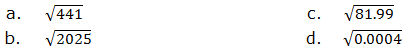Home MATHEMATICS TOPIC 1: EXPONENTS AND RADICALS ~ MATHEMATICS FORM TWO

# TOPIC 1: EXPONENTS AND RADICALS ~ MATHEMATICS FORM TWO

2083
0Exponents

Exponents tell how many times to use a number itself in multiplication. There are different laws that guides in calculations involving exponents. In this chapter we are going to see how these laws are used.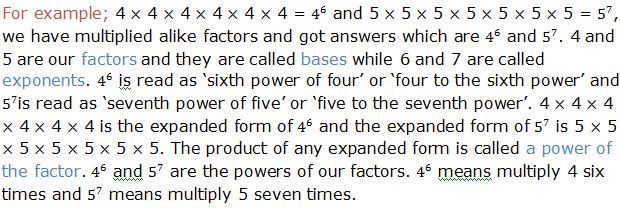Indication of power, base and exponent is done as follows: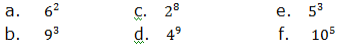Solution: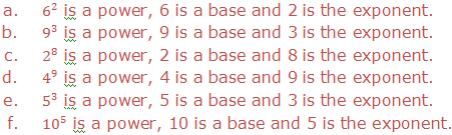To write the expanded form of the following powers: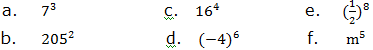Solution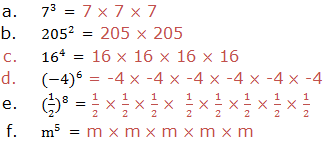To write each of the following in power form: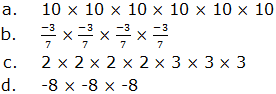Soln.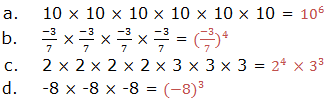The Laws of Exponents

List the laws of exponents

First law:Multiplication of positive integral exponent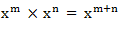Second law: Division of positive integral exponent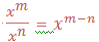Third law: Zero exponents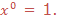Fourth law: Negative integral exponents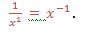Verification of the Laws of Exponents

Verify the laws of exponents

First law: Multiplication of positive integral exponent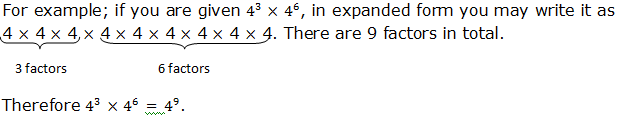Generally,
when we multiply powers having the same base, we add their exponents.
If x is any base and m and n are the exponents, therefore:Example 1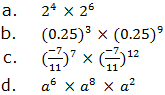SolutionIf you are to write the expression using the single exponent, for example,(63)4.The expression can be written in expanded form as: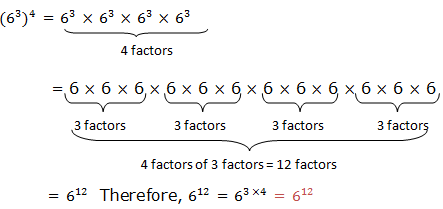Generally if a and b are real numbers and n is any integer,Example 2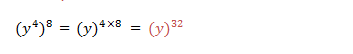Example 3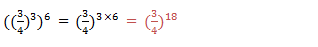Example 4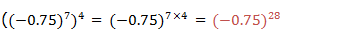Generally, (xm)= X(mxn)
Example 5

Rewrite the following expressions under a single exponent for those with identical exponents: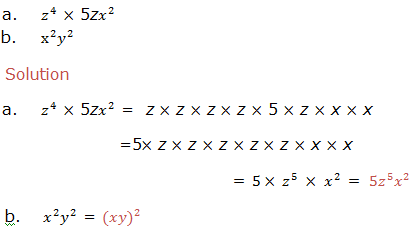Second law: Division of positive integral exponent
Example 6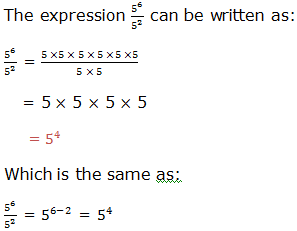Example 7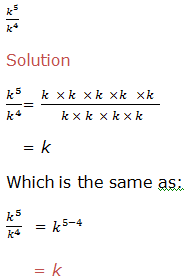Therefore,
to divide powers of the same base we subtract their exponents (subtract
the exponent of the divisor from the exponent of the dividend). That
is,where x is a real number and x ≠ 0, m and n are integers. m is the exponent of the dividend and n is the exponent of the divisor.

Example 8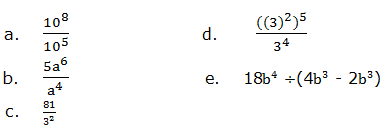solution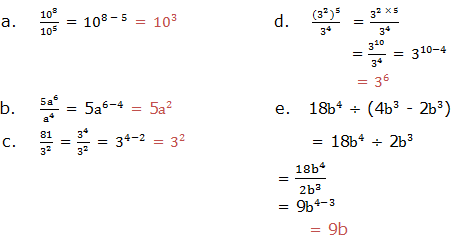Third law: Zero exponents
Example 9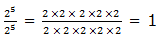This is the same as: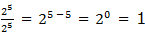If a ≠ 0, then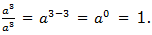Which is the same as: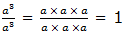Therefore if x is any real number not equal to zero, then X0 = 1,Note that 00is undefined (not defined).
Fourth law: Negative integral exponents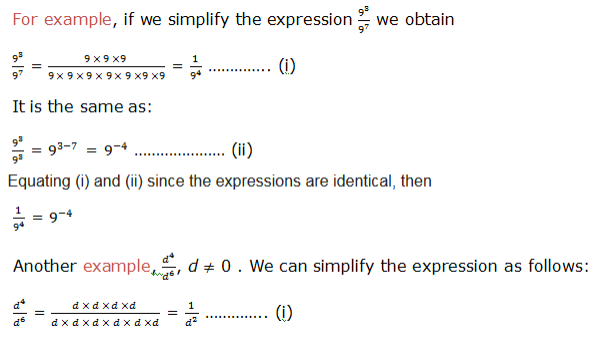Also;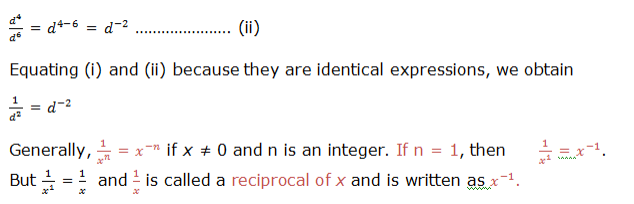Example 10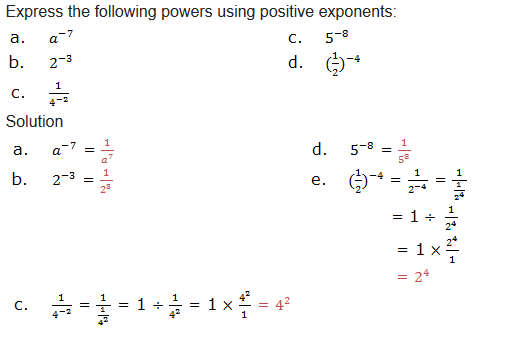Exercise 1
1. Indicate base and exponent in each of the following expressions: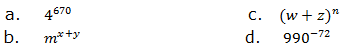2. Write each of the following expressions in expanded form: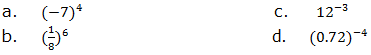3. Write in power form each of the following numbers by choosing the smallest base:
1. 169
2. 81
3. 10,000
4. 625
a. 169 b. 81c. 10 000 d. 625
4. Write each of the following expressions using a single exponent: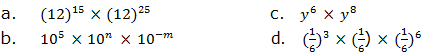5. Simplify the following expressions: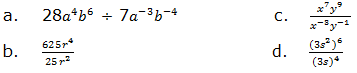6. Solve the following equations: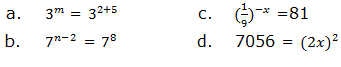7. Express 64 as a power with:
1. Base 4
2. Base 8
3. Base 2
Base 4 Base 8 Base 2
8. Simplify the following expressions and give your answers in either zero or negative integral exponents.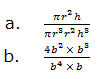9. Give the product in each of the following: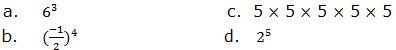10. Write the reciprocal of the following numbers: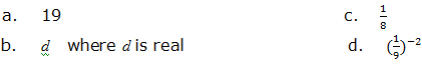Laws of Exponents in Computations
Apply laws of exponents in computations
Example 11SolutionRe-arranging Letters so that One Letter is the Subject of the Formula
Re-arrange letters so that one letter is the subject of the formula

A formula is a rule which is used to calculate one quantity when other quantities are given. Examples of formulas are: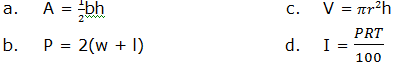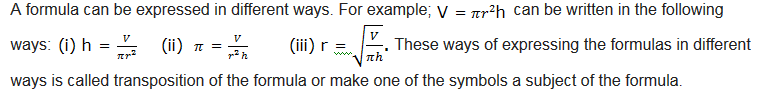Example 14
From the following formulas, make the indicated symbol a subject of the formula: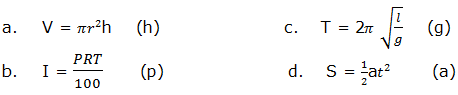Solution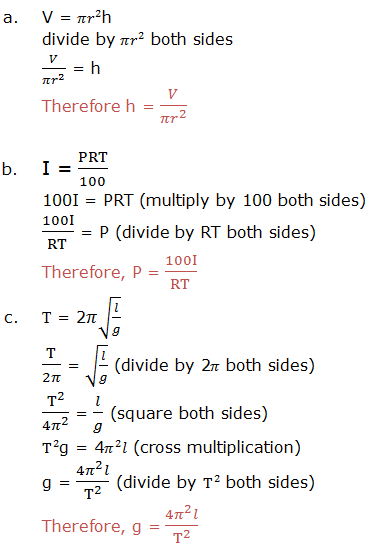Transposing a Formulae with Square Roots and Square

Transpose a formulae with square roots and square

Make the indicated symbol a subject of the formula: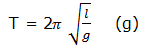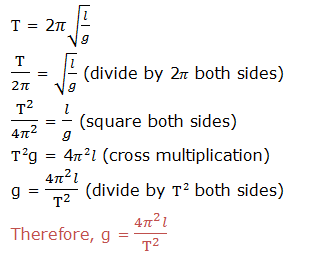Exercise 3

#### 1. Change the following formulas by making the given letter as the subject of the formula.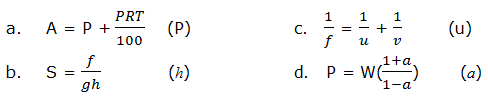2. Use mathematical tables to find square root of each of the following: